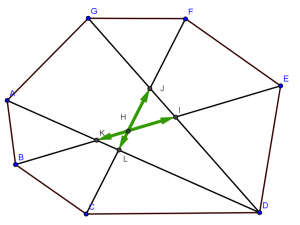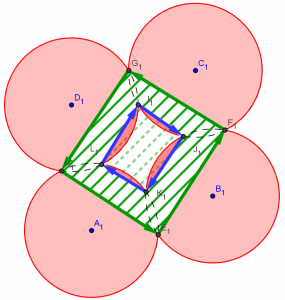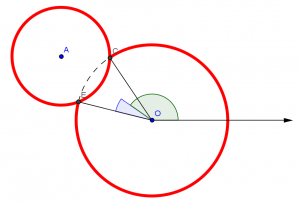# 线性时间内筛素数和欧拉函数

## 算法的实质

S = {2, ..., n}
for (p = 2; p*p <= n; p = next(S, p)):
print p
for (i = p; i*p <= n; i = next(S, i)):
for (j = i*p; j <= n; j = j*p):
S = S - {j}

next(S, i) is a function defined only for i ∈ S such that
there is an integer larger than i in S; it yields the next
larger integer in S. 

# [POJ3743]LL’s Cake

## 求域# [POJ3017]Cut the Sequence

f[i] = min{ f[j] + max{a[j+1]..a[i]} } (sum[i]-sum[j] <= M)

# [SPOJ CIRUT]CIRU2

• 原先做线段覆盖，现在改为括号匹配（压栈）。原先计算没被覆盖的面积，现在计算至少覆盖k+1次的面积area[k+1]，答案就是area[k]-area[k+1]
• 如果一个圆被完全包含，原先直接扔掉，现在要加上去。

# [SPOJ CIRU]The Area of the Union of Circles

AC大神写过一篇圆面积并的文章，好像大家都是从他那边学来的（吐槽一下百度，自家空间的内容搜不到，但是谷歌就可以）：求圆并的若干种算法,圆并扩展算法

AC大神提出了一种很厉害的方法：对于每个圆，枚举所有和它相交的圆，然后记下所有相交的区间，剩下的区间（若干条弧），计算弧所对应的弓形还有弧所对应的有向线段的有向面积。# [BZOJ1791][IOI2008]Island 岛屿

## 分析

• 两端在某一棵外向树上
• 一端在某一棵外向树上，然后走了环的一部分，最终到达另外一棵外向树

# [BZOJ1043][HAOI2008]下落的圆盘